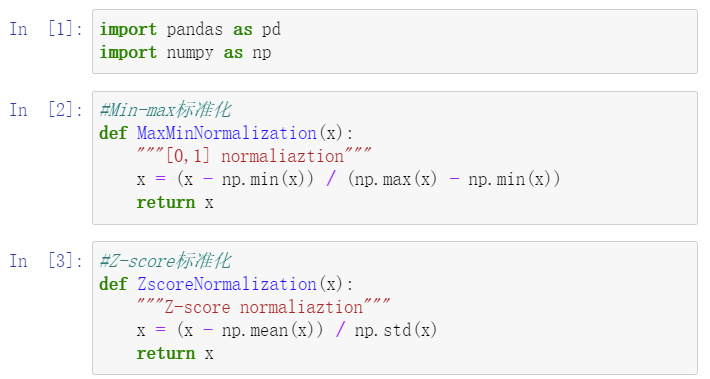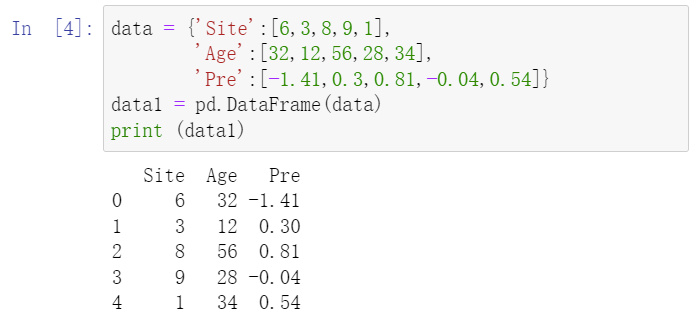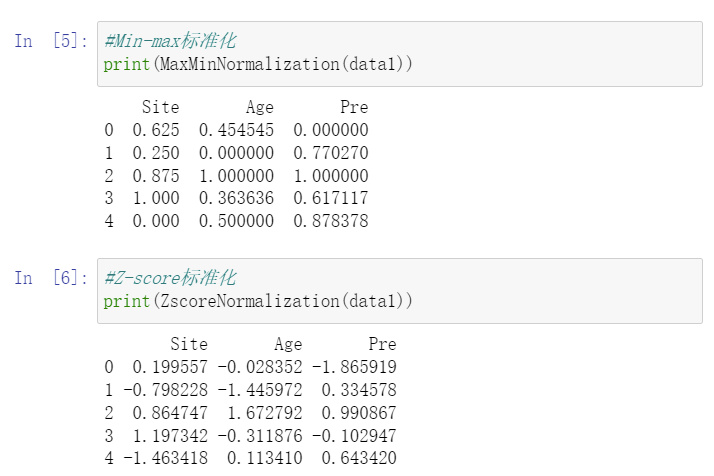• python数据标准化
千次阅读
2021-03-12 14:56:15
# 导入归一化方法
from sklearn.preprocessing import MinMaxScaler
mms = MinMaxScaler()

# 调用方法对数据进行归一化处理

data['X_train'] = mms.fit_transform(data['X_train'])

X_train ：需要做归一化处理的数据列、或者数据框

2  正态标准化

使用环境：线性回归需要数据呈现正态分布趋势、其他的聚类等及机器学习的场景

from sklearn.preprocessing import StandardScaler

stdsc=StandardScaler()

iris_data=stdsc.fit_transform(iris_data)
iris_data：需要做正态标准化处理的数据列、或者数据框

————————————————
版权声明：本文为CSDN博主「UP Lee」的原创文章，遵循CC 4.0 BY-SA版权协议，转载请附上原文出处链接及本声明。

> 原文链接：https://blog.csdn.net/qq_36327687/article/details/84657570python
更多相关内容
• ## Python数据标准化

千次阅读 2022-03-17 21:39:48
数据标准化

## 1.最小最大标准化

def MinMaxNorm(x):
# 标准化
min_val = np.nanmin(x, axis=0)
max_val = np.nanmax(x, axis=0)
for i in range(len(x)):
x[i] = (x[i] - min_val) / (max_val - min_val)
return x


## 2.Z-Score标准化

# 标准化
def robust_zscore(x: pd.Series, zscore=False):
# https://en.wikipedia.org/wiki/Median_absolute_deviation
# 删除以异常值
x = x - x.median()
x = np.clip(x / mad / 1.4826, -3, 3)

# 标准化
if zscore:
x -= x.mean()
x /= x.std()
return x

展开全文python
• 本文为大家分享了python数据分析数据标准化及离散化的具体内容，供大家参考，具体内容如下 标准化 1、离差标准化 是对原始数据的线性变换，使结果映射到[0,1]区间。方便数据的处理。消除单位影响及变异大小因素影响...
• ## python数据标准化

千次阅读 2017-10-25 10:33:16
def datastandard(): from sklearn import preprocessing import numpy as np x = np.array([ [ 1., -1., 2.], [ 2., 0., 0.], [ 0., 1., -1.]]) print('原始数据为：\n',x) print('method1:指定...
def datastandard():
from sklearn import preprocessing
import numpy as np
x = np.array([
[ 1., -1.,  2.],
[ 2.,  0.,  0.],
[ 0.,  1., -1.]])
print('原始数据为：\n',x)

print('method1:指定均值方差数据标准化(默认均值0 方差 1):')
print('使用scale()函数 按列标准化')
x_scaled = preprocessing.scale(x)
print('标准化后矩阵为:\n',x_scaled,end='\n\n')
print('cur mean:', x_scaled.mean(axis=0), 'cur std:', x_scaled.std(axis=0))

print('使用scale()函数 按行标准化')
x_scaled = preprocessing.scale(x,axis=1)
print('标准化后矩阵为:\n',x_scaled,end='\n')
print('cur mean:', x_scaled.mean(axis=1), 'cur std:', x_scaled.std(axis=1))

print('\nmethod2:StandardScaler类,可以保存训练集中的参数')
scaler = preprocessing.StandardScaler().fit(x)
print('标准化前 均值方差为:',scaler.mean_,scaler.scale_)
print('标准化后矩阵为:\n',scaler.transform(x),end='\n\n')

print('***2.数据归一化,映射到区间[min,max]：')
min_max_scaler = preprocessing.MinMaxScaler(feature_range=(0,10))
print(min_max_scaler.fit_transform(x))

if __name__ == '__main__':
datastandard()


结果如下：

原始数据为：
[[ 1. -1.  2.]
[ 2.  0.  0.]
[ 0.  1. -1.]]
method1:指定均值方差数据标准化(默认均值0 方差 1):
使用scale()函数 按列标准化
标准化后矩阵为:
[[ 0.         -1.22474487  1.33630621]
[ 1.22474487  0.         -0.26726124]
[-1.22474487  1.22474487 -1.06904497]]
cur mean: [ 0.  0.  0.] cur std: [ 1.  1.  1.]

使用scale()函数 按行标准化
标准化后矩阵为:
[[ 0.26726124 -1.33630621  1.06904497]
[ 1.41421356 -0.70710678 -0.70710678]
[ 0.          1.22474487 -1.22474487]]
cur mean: [  1.48029737e-16   7.40148683e-17   0.00000000e+00] cur std: [ 1.  1.  1.]

method2:StandardScaler类,可以保存训练集中的参数
标准化前 均值方差为: [ 1.          0.          0.33333333] [ 0.81649658  0.81649658  1.24721913]
标准化后矩阵为:
[[ 0.         -1.22474487  1.33630621]
[ 1.22474487  0.         -0.26726124]
[-1.22474487  1.22474487 -1.06904497]]

***2.数据归一化,映射到区间[min,max]：
[[  5.           0.          10.        ]
[ 10.           5.           3.33333333]
[  0.          10.           0.        ]]


### python 常用代码

# coding:utf8
'''
提取文档中含有某个字符的所有行，并打印出来
'''
file_path = 'E:/gengyanpeng/keyun-bi.sql'
fix_str = 'FROM'

def print_line(txt,fix_str):
lines = txt.split('\n')
for line in lines:
if fix_str in line:
print(line.strip())

with open(file_path,'r+',encoding='utf8') as f:

print_line(text,fix_str)

展开全文python
• 【小白从小学Python、C、Java】 【Python全国计算机等级考试】 【Python数据分析考试必会题】 ● 标题与摘要 Python数据分析中的 数据预处理：数据标准化 ...1.数据标准化是什么：在数据分析之前，我们通常

【小白从小学Python、C、Java】

【Python全国计算机等级考试】

【Python数据分析考试必会题】

● 标题与摘要

Python数据分析中的

数据预处理：数据标准化

● 选择题

以下关于数据标准化说法错误的是：

A 将数据按比例缩放，使之落入一个小的特定区间

B Min-max标准化是数据标准化的方法

C Z-score标准化是数据标准化的方法

D Min-max标准化后结果落到[-1,1]区间

​● 问题解析

1.数据标准化是什么：在数据分析之前，我们通常需要先将数据标准化，利用标准化后的数据进行数据分析。数据的标准化是将数据按比例缩放，使之落入一个小的特定区间。常用的方法有Min-max标准化，Z-score标准化。A，B，C正确

2.数据标准化的作用：数据标准化处理主要包括数据同趋化处理和无量纲化处理两个方面。数据同趋化处理主要解决不同性质（正指标和逆指标）的数据问题，对不同性质指标直接加总不能正确反映不同指标带来的综合结果，须先考虑改变逆指标数据性质，使所有指标对测评方案的作用力同趋化，再加总才能得出正确结果。数据无量纲化处理主要解决数据的可比性。

3.Min-max标准化是最基本的一种数据标准化方法，指的是将数据压缩到[0,1]之间。标准化的公式为：新数据=（原数据-最小值）/（最大值-最小值）。（说明：最小值，最大值为原数据的最小值，最大值）。D错误

4.Z-score标准化基于原始数据的均值（mean）和标准差（standard deviation）进行数据的标准化。标准化的公式为：新数据=（原数据-均值）/标准差。（说明：均值，标准差为原数据的均值，标准差）。

​● 附图

图1 用python实现两种标准化方法——写数据标准化的函数图2 原数据展示图3 用python实现两种标准化方法——对数据进行标准化● 附图代码

import pandas as pd

import numpy as np

#Min-max标准化

def MaxMinNormalization(x):

"""[0,1] normaliaztion"""

x = (x - np.min(x)) / (np.max(x) - np.min(x))

return x

#Z-score标准化

def ZscoreNormalization(x):

"""Z-score normaliaztion"""

x = (x - np.mean(x)) / np.std(x)

return x

data = {'Site':[6,3,8,9,1],

'Age':[32,12,56,28,34],

'Pre':[-1.41,0.3,0.81,-0.04,0.54]}

data1 = pd.DataFrame(data)

print (data1)

#Min-max标准化

print(MaxMinNormalization(data1))

#Z-score标准化

print(ZscoreNormalization(data1))

● 正确答案

D

欢迎大家转发，一起传播知识和正能量，帮助到更多人。期待大家提出宝贵改进建议，互相交流，收获更大。辛苦大家转发时注明出处(也是咱们公益编程交流群的入口网址)，刘经纬老师共享知识相关文件下载地址为：http://liujingwei.cn

展开全文python 数据挖掘
•python
• 1.数据标准化含义：将数据的数值特征转换成一些标准的格式，使其与其他属性处于相同的范围内，此过程叫标准化（Normalization） 2.有两种常用的标准化技术 最大最小标准化（Min-Max Normalization）:这个过程使得...python
• 数据标准化：是指将数据按比例缩放，使之落入到特定区间   1 进行0-1 表转化计算公式 使用环境： 在聚类分析，主成分分析的时候，因为量纲不一所以需要 # 导入归一化方法 from sklearn.preprocessing import ...数据标准化处理 正态化
• 在机器学习过程中，对数据的处理过程中，常常需要对数据进行归一化处理，下面介绍（0， 1）标准化的方式，简单的说，其功能就是将预处理的数据的数值范围按一定关系“压缩”到（0，1）的范围类。 通常（0， 1）标注...
• 本文实例讲述了Python数据预处理之数据规范化。分享给大家供大家参考，具体如下： 数据规范化 为了消除指标之间的量纲和取值范围差异的影响，需要进行标准化（归一化）处理，将数据按照比例进行缩放，使之落入一个...
• 零一标准化 零一标准化 import pandas as pd import matplotlib.pyplot as mp d = {'name': list('ABCD'), 'value': [1002, 1000, 1010, 1001]} df = pd.DataFrame(d) # 0-1标准化 value...python 01标准化
• 下面是一些常用的归一化、标准化的方法： 离差标准化 log归一化 标准化 比例归一化 反正切归一化 代码示例 import numpy as np def min_max_normalization(value): """归一化，也称离差标准化 公式：(原始值-...python
• StandardScaler类是一个用来讲数据进行归一化和标准化的类。-计算训练集的平均值和标准差，以便测试数据集使用相同的变换。样本x的标准分数计算如下：z = (x - u) / s将数据按期属性(按列进行)删除平均值和缩放到...python数据标准
• # 标准化（Z-Score）(X-mean)/std data1_scaled = preprocessing.scale(data1) data2=pd.DataFrame(data1_scaled,columns=[ 'C' , 'F' , 'L' , 'M' , 'R' ]) print data2 # ######pandas写出excel data1.to_...python
• 什么是数据标准化(归一化)数据标准化(归一化)处理是数据挖掘的一项基础工作，不同评价指标往往具有不同的量纲和量纲单位，当各指标间的水平相差很大时，如果直接用原始指标值进行分析，就会突出数值较高的指标在综合...
• 数据标准化处理 问题： 量纲不一：就是单位、特征的单位不一致，不能放在一起比较 处理方法： 0-1标准化、Z标准化、normalizer归一化 案例： # -*- coding: utf-8 -*- import pandas import numpy data=...数据归一化 Z标准化
• <p></p><div class="toc"><h3>文章目录</h3><ul><ul><ul><ul><li>数据标准化</a></li><li><a href="#_7" rel="nofollow" data-token="cd47b6def2f5b8901521d02b02d6f57a" target="_self">常见的几种形式</a></li>...
• 这篇文章主要介绍了python数据预处理之数据标准化的几种处理方式，文中通过示例代码介绍的非常详细，对大家的学习或者工作具有一定的参考学习价值，需要的朋友们下面随着小编来一起学习学习吧 何为标准化： 在数据...python 编程语言
• 含义数据标准化和归一化存在区别数据归一化是数据标准化的一种典型做法,即将数据统一映射到[0,1]区间上.数据的标准化是指将数据按照比例缩放,使之落入一个特定的区间.意义求解需要比如在SVM中处理分类问题是又是需要...
• ## 数据标准化处理

千次阅读 2020-12-05 20:57:00
正态分布跟标准分数之间的关系Python版本：3.5数学公式编辑器：MathType标准分数标准分数(Standard Score，又称z-score、z分数或标准化值)，是将集合中的单个数与集合的均值相减的结果除以集合的标准差得到标准化的...
• Python sklearn学习之数据预处理——标准化 文章目录Python sklearn学习之数据预处理——标准化1. 数据集常见标准化方式min-max标准化(Min-... 数据标准化实现2.1 z-score 标准化(zero-mean-normalization)2.1.1 Sta...机器学习 Python sklearn
• 对一个一维数组进行了离差标准化，讲数组数据归一化，进而进行下一步神经网络的建模。python
• 一、数据标准化（归一化） 首先，数据标准化处理主要包括数据同趋化处理（中心化处理）和无量纲化处理。同趋化处理主要解决不同性质数据问题，对不同性质指标直接加总不能正确反映不同作用力的综合结果，须先考虑...
• 本文使用python实现max-max和z-score两种标准化方法 import pandas as pd import numpy as np test_dict = {'id':[1,2,3,4,5,6], 'num':[1,2,1,1,4,5], 'total':[54,49,51,52,51,50] } test_df = pd.DataFrame...
• 今天小编就为大家分享一篇使用sklearn进行对数据标准化、归一化以及将数据还原的方法，具有很好的参考价值，希望对大家有所帮助。一起跟随小编过来看看吧在对模型训练时，为了让模型尽快收敛，一件常做的事情就是对...python 人工智能 编程语言
• 标准化和规范化是机器学习和深度学习项目中大量使用的数据预处理技术之一。 这些技术的主要作用 以类似的格式缩放所有数据，使模型的学习过程变得容易。 数据中的奇数值被缩放或归一化并且表现得像数据的一部分。 ...机器学习 标准化 sklearn
• 在数据分析与挖掘、算法建模的都会用到数据标准化。数据的标准化(normalization)是将数据按比例缩放，使之落入一个小的特定区间。在某些比较和评价的指标处理中经常会用到，去除数据的单位限制，将其转化为无量纲的...数据标准化 Python
• 数据聚焦于数据的采集、清理、预处理、分析和挖掘，图形聚焦于解决对光学图像进行接收、提取信息、加工变换、模式识别及存储显示，可视聚焦于解决将数据转换成图形，并进行交互处理。 信息：是数据的内涵，信息是...python...